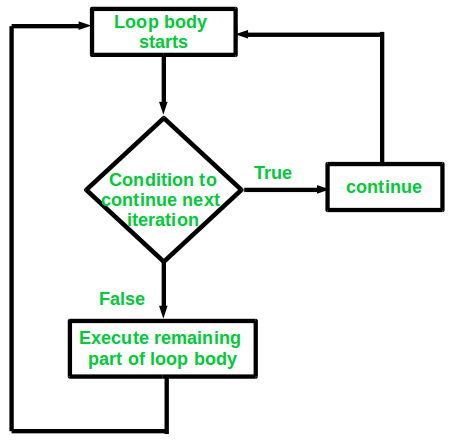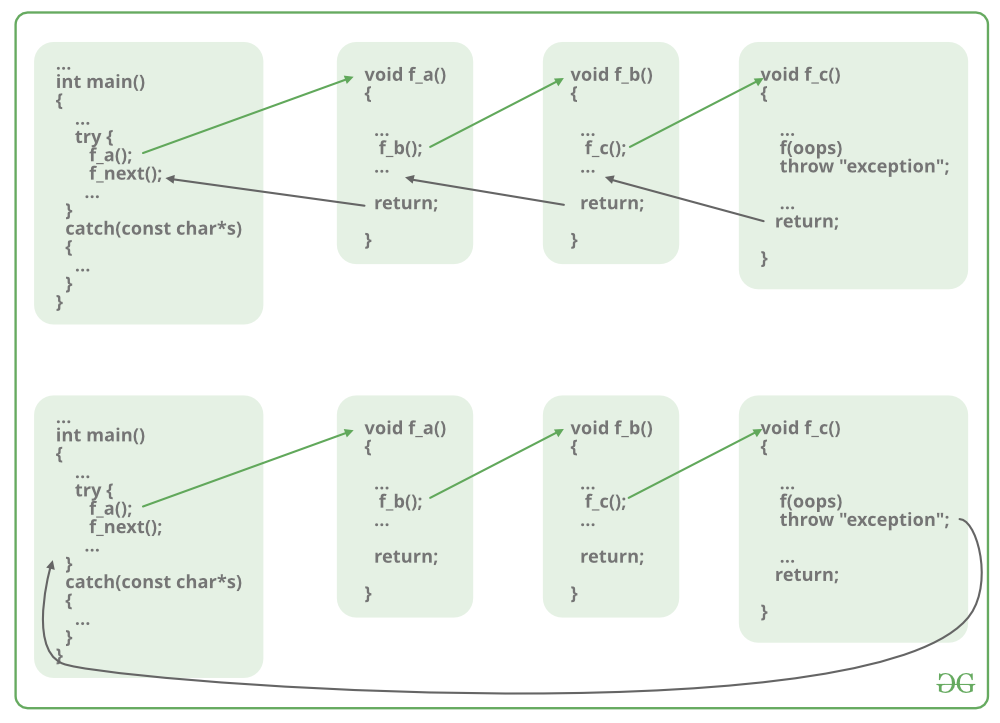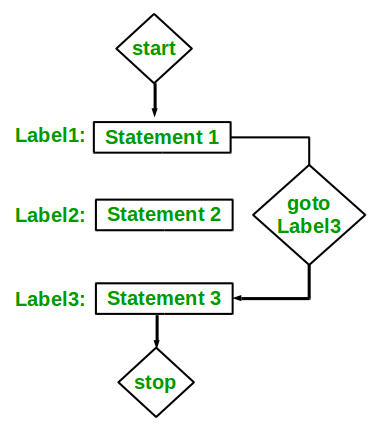Related Articles

# Jump statements in C++

• Difficulty Level : Basic
• Last Updated : 13 Jan, 2021

Jump statements are used to manipulate the flow of the program if some conditions are met. It is used to terminating or continues the loop inside a program or to stop the execution of a function.  In C++ there is four jump statement: continue, break, return, and goto

Continue: It is used to execute other parts of the loop while skipping some parts declared inside the condition, rather than terminating the loop, it continues to execute the next iteration of the same loop. It is used with a decision-making statement which must be present inside the loop. This statement can be used inside for loop or while or do-while loop.Syntax:

`continue;`

Program 1:

Consider a scenario where all the numbers between 1 and 10 except number 5. So in this case, the idea is to use the continue statement after the value of i is 5. Below is the program for the same:

## C++

 `// C++ program to demonstrate the``// continue statement``#include ``using` `namespace` `std;`` ` `// Driver code``int` `main()``{``    ``for` `(``int` `i = 1; i < 10; i++) {`` ` `        ``if` `(i == 5)``            ``continue``;``        ``cout << i << ``" "``;``    ``}``    ``return` `0;``}`
Output:
```1 2 3 4 6 7 8 9
```

Break: It is used to terminate the whole loop if the condition is met. Unlike the continue statement after the condition is met, it breaks the loop and the remaining part of the loop is not executed. Break statement is used with decision-making statements such as if, if-else, or switch statement which is inside the for loop which can be for loop, while loop, or do-while loop. It forces the loop to stop the execution of the further iteration.Syntax:

`break;`

Program 2:

Consider a scenario where the series of a number is to be printed but not after a certain value K. So in this case, the idea is to use the break statement after the value of i is K. Below is the program for the same:

## C++

 `// C++ program to demonstrate the``// break statement``#include ``using` `namespace` `std;`` ` `// Driver Code``int` `main()``{``    ``for` `(``int` `i = 1; i < 10; i++) {`` ` `        ``// Breaking Condition``        ``if` `(i == 5)``            ``break``;``        ``cout << i << ``" "``;``    ``}``    ``return` `0;``}`
Output:

```1 2 3 4
```

Return: It takes control out of the function itself. It is stronger than a break. It is used to terminate the entire function after the execution of the function or after some condition. Every function has a return statement with some returning value except the void() function. Although void() function can also have the return statement to end the execution of the function.Syntax:

`return expression;`

Program 3:

Below is the program to demonstrate the return statement:

## C++

 `// C++ program to demonstrate the``// return statement``#include ``using` `namespace` `std;`` ` `// Driver code``int` `main()``{``    ``cout << ``"Begin "``;`` ` `    ``for` `(``int` `i = 0; i < 10; i++) {`` ` `        ``// Termination condition``        ``if` `(i == 5)``            ``return` `0;``        ``cout << i << ``" "``;``    ``}``    ``cout << ``"end"``;`` ` `    ``return` `0;``}`
Output:
```Begin 0 1 2 3 4
```

Explanation:

The above program starts execution by printing “Begin” then the for loop starts to print the value of, it will print the value of i from 0 to 4 but as soon as i becomes equal to 5 it will terminate the whole function i.e., it will never go to print the “end” statement of the program.

The return in void() functions can be used without any return type.

Syntax:

`return;`

Program 5:

Below is the program to demonstrate the return statement in void return type in function:

## C++

 `// C++ program to demonstrate the return``// statement in void return type function``#include ``using` `namespace` `std;`` ` `// Function to find the greater element``// among x and y``void` `findGreater(``int` `x, ``int` `y)``{``    ``if` `(x > y) {``        ``cout << x << ``" "``             ``<< ``"is greater"``             ``<< ``"\n"``;``        ``return``;``    ``}``    ``else` `{``        ``cout << y << ``" "``             ``<< ``"is greater"``             ``<< ``"\n"``;``        ``return``;``    ``}``}`` ` `// Driver Code``int` `main()``{``    ``// Function Call``    ``findGreater(10, 20);`` ` `    ``return` `0;``}`
Output:
```20 is greater
```

Goto: This statement is used to jump directly to that part of the program to which it is being called.  Every goto statement is associated with the label which takes them to part of the program for which they are called. The label statements can be written anywhere in the program it is not necessary to use before or after goto statement. This statement makes it difficult to understand the flow of the program therefore it is avoided to use it in a program.Syntax:

```goto label_name;
.
.
.
label_name:
```

Program 6:

Below is the program to demonstrate the goto statement:

## C++

 `// C++ program to demonstrate the``// goto statement``#include ``using` `namespace` `std;`` ` `// Driver Code``int` `main()``{``    ``int` `n = 4;`` ` `    ``if` `(n % 2 == 0)``        ``goto` `label1;``    ``else``        ``goto` `label2;`` ` `label1:``    ``cout << ``"Even"` `<< endl;``    ``return` `0;`` ` `label2:``    ``cout << ``"Odd"` `<< endl;``}`
Output:
```Even
```

Explanation: The above program is used to check whether the number is even or odd if the number pressed by the user says it is 4 so the condition is met by the if statement and control go to label1 and label1 prints that the number is even. Here it is not necessary to write a label statement after the goto statement we can write it before goto statement also it will work fine.

Want to learn from the best curated videos and practice problems, check out the C++ Foundation Course for Basic to Advanced C++ and C++ STL Course for foundation plus STL.  To complete your preparation from learning a language to DS Algo and many more,  please refer Complete Interview Preparation Course.

My Personal Notes arrow_drop_up